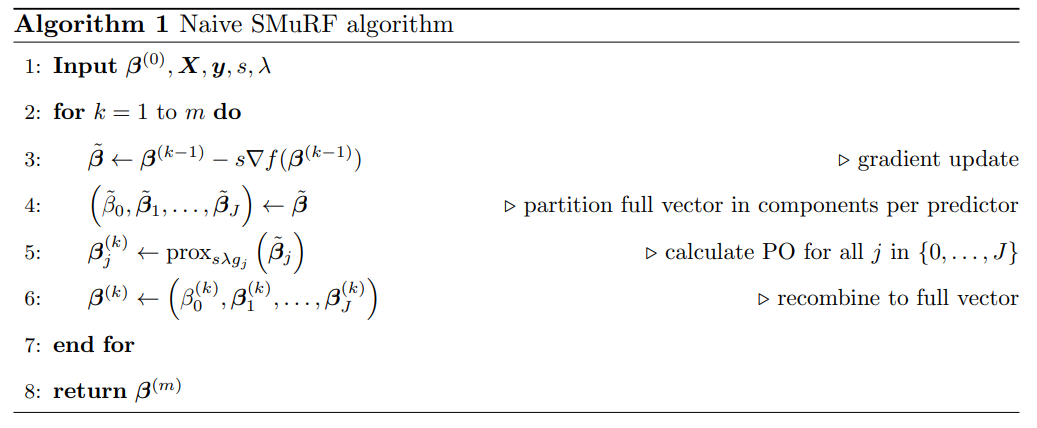# Sparse Regression with Multi-type Regularized Feature Modeling### Abstract

Within the statistical and machine learning literature, regularization techniques are often used to construct sparse (predictive) models. Most regularization strategies only work for data where all predictors are treated identically, such as Lasso regression for (continuous) predictors treated as linear effects. However, many predictive problems involve different types of predictors and require a tailored regularization term. We propose a multi-type Lasso penalty that acts on the objective function as a sum of subpenalties, one for each type of predictor. As such, we allow for predictor selection and level fusion within a predictor in a data-driven way, simultaneous with the parameter estimation process. We develop a new estimation strategy for convex predictive models with this multi-type penalty. Using the theory of proximal operators, our estimation procedure is computationally efficient, partitioning the overall optimization problem into easier to solve subproblems, specific for each predictor type and its associated penalty. Earlier research applies approximations to non-differentiable penalties to solve the optimization problem. The proposed SMuRF algorithm removes the need for approximations and achieves a higher accuracy and computational efficiency. This is demonstrated with an extensive simulation study and the analysis of a case-study on insurance pricing analytics.

Type
Publication
Insurance: Mathematics and Economics, 91, 248-261.